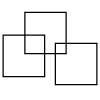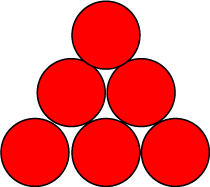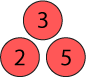#### You may also like### Three Squares

What is the greatest number of squares you can make by overlapping three squares?### Two Dice

Find all the numbers that can be made by adding the dots on two dice.### Biscuit Decorations

Andrew decorated 20 biscuits to take to a party. He lined them up and put icing on every second biscuit and different decorations on other biscuits. How many biscuits weren't decorated?

# Find the Difference

## Find the Difference

Place the numbers $1$ to $6$ in the circles so that each number is the difference between the two numbers just below it.Example:$5 - 2 = 3$

You could try it in this interactivity.

When you have tried this and got more than one answer you could try other things like having four rows of discs and use the numbers $1$ to $10$.

### Why do this problem?

This problem is a challenging way of practising subtraction at the same time as being logical about arranging the numbers. The idea of 'difference' can be hard for children to grasp and this problem is an ideal way of coming to terms with it. You could also use this problem to focus on how children record their workings.

### Possible approach

You could start by putting the numbers in any places in the pyramid and asking children to describe what they see. They may notice some accidental number patterns, but also which numbers are used. Then put two numbers into the pyramid, for example $4$ and $5$ in the bottom row, next to each other. Introduce the idea of the problem and invite pupils to suggest what number should go above the $4$ and $5$. You could repeat this a few times with different pairs of numbers so that children are happy with 'difference' and with the way the pyramid will be structured. You may need to emphasise that the smaller number will be taken away from the larger number each time.

Introduce the task as stated in the problem and encourage learners to work in pairs. They may want to use the interactivity on computers and/or they could work on paper, using this sheet of blank pyramids for rough working and recording. If you want a whole class to work on the problem at the same time, this sheet gives the pyramid of circles, the numbers from 1 to 6 to cut out and leaves space for adding another row of circles should this be required. After a short time, it would be useful to encourage learners to share the ways they are working and what they are recording. Some might be trying numbers then adjusting them, others might have thought about where, for example, the largest must go, others might be taking one number at a time and looking at the different places it could go in turn. The key point to make is that their recording system should somehow enable them to keep track of what arrangements they have tried so far so they don't repeat themselves. It is an advantage, therefore, not to use an eraser!

The plenary can be used to compare different solutions, using the interactivity, and to discuss the advantages of the different recording methods.

### Key questions

Where could the largest number go? Why?
What do we need to do to find the difference between two numbers?

### Possible extension

Some children could be challenged to find as many different arrangements as possible.
They might also like to try this very challenging problem which uses the numbers from 1 - 10.

### Possible support

Some children would benefit from using numbered counters that can be moved about if they don't have access to the interactivity. Some children may need to use manipulatives to support them in finding the differences.